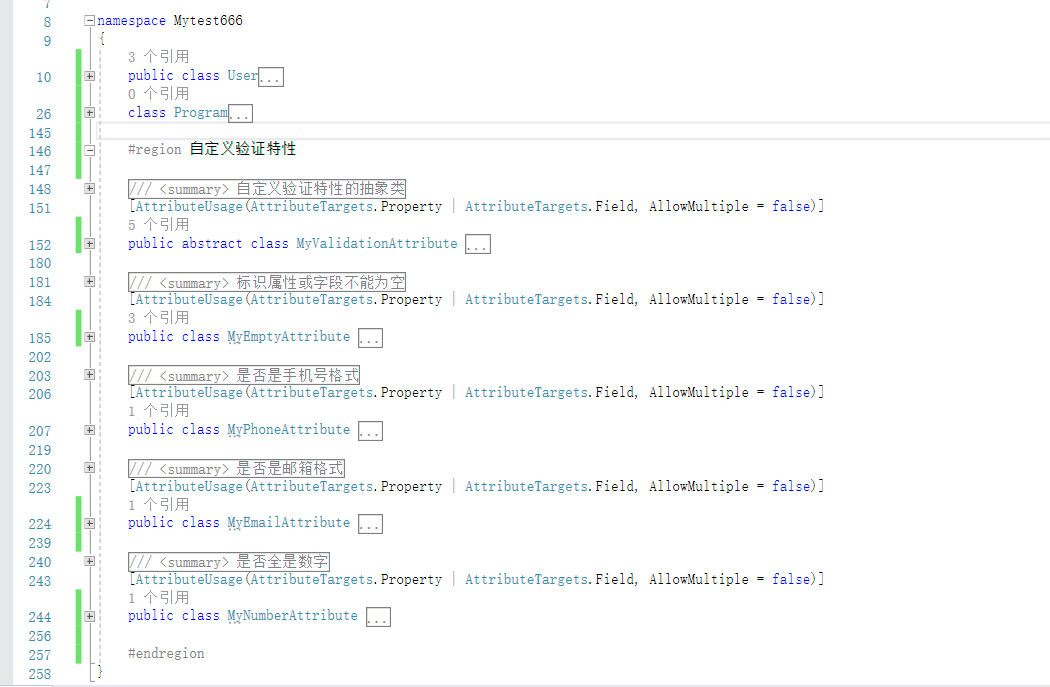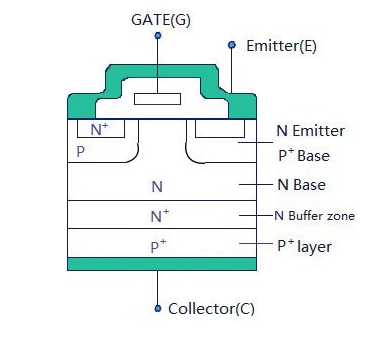+关注继续查看

# 一、nullptr

1 int fun(char* ch){}
2 int fun(int num){}

# 二、constexpr

constexpr变量必须是一个常量，必须用常量表达式来初始化，例如下面代码

1 const int a = 10;
2 int b = 10;
3 constexpr int d = 10;  //正确
4 constexpr int c = a + 10;  //正确
5 constexpr int e = b;  //错误

值得一提的是如果成员函数标记为constexpr，则默认其是内联函数，如果变量声明为constexpr，则默认其是const，这个会在构造函数中用到。

# 三、类型推导

## auto

1 map<int,int>::iterator it = mp.begin();
2 auto it = mp.begin();

1 int fun(int i){
2     cout << i << endl;
3 }
4
5 function<int(int)> f;
6 auto a = fun;

auto fun(int i){
}

## decltype

    auto a = 1,b = 2;
decltype(a+b) z;

## 拖尾返回类型

1 template<typename R, typename T, typename U>
2 R add(T x, U y) {
3     return x+y
4 }

当有多个这种函数的时候，就会显得代码很冗长，很不人性化qwq，所以就出现了拖尾返回类型，例如下面这个代码

1 auto fun(int i) -> bool{
2     return i&1;
3 }

1 template<typename T>
2 auto fun(T i) -> decltype(i*i){
3     return i*i;
4 }

# 四、区间迭代

1 for(vector<int>::iterator iter = vec.begin(); iter != vec.end(); iter++)

1 for(auto &iter : vec)

# 五、尖括号'>'

1 vector<vector<int>> q;

# 六、初始值

1 struct node{
2     int a,b;
3     node(int _a,int _b){this->a = _a,this->b = _b;}
4     node(int _a,int _b):a(_a),b(_b){}
5 };

1 struct node{
2     int a,b;
3 };
4
5 node asd{1,2};
6 node as = {1,2};

# 七、类型别名以及默认别名

## 类型别名

typedef大家肯定使用过，一般将一个类或者结构体做别名，但对于容器，比如下面用法

1 template<typename T>
2 typedef vector<T> qwq;

1 template<typename T>
2 using asd = vector<T>;

1 asd<long long> vec;

## 默认别名

1 template<typename T1,typename T2>
2 auto fun(T1 a,T2 b)->bool{
3     return a < b;
4 }

1 template<typename T1 = int,typename T2 = int>
2 auto fun(T1 a,T2 b)->bool{
3     return a < b;
4 };

# 八、lambda表达式

Lambda表达式是用来创建匿名函数的。什么叫做匿名函数，就是没有名字qwq，那么为什么要用到匿名函数呢，举个例子，比如sort的第三个参数，谓词函数，一般我们会写一个比较函数，但这样的后果就是，如果有多个sort你就得，每个函数去找他的比较函数，实属麻烦。各位看下面这个例子

1 sort(a.begin(),a.end(),[](int a,int b)->bool{return a < b;});

1 [capture]（parameters）opt->return-type{body}

emmm 后面那一截和普通函数没什么区别，参数，返回值类型，函数体。和函数最大的不同就是匿名函数是临时函数，没有函数名，及拿及用，当然你也可以用function保存一个匿名函数。

1.返回值类型->return-type可以省略，由语言自动推导，但只限于lambda表达式语句简单。

2.引入lambda表达式的前导符是一对方括号，叫做lambda引入符。lambda表达式可以使用与其相同范围内的变量，一共有下面这么几种方式捕获变量

[]//不捕获任何外部变量

[=]//以值形式捕获所有外部变量

[&]//以引用方式捕获所有外部变量

[x,&y]//x以值形式捕获，y以引用形式捕获

[=,&x]//x以引用形式捕获，其余变量以值捕获

[&,x]//x以值形式捕获，其余变量以引用形式捕获

[this]捕获当前类的指针。捕获this的目的是可以在lambda中使用当前类的成员函数和成员变量。

3.opt函数选项

mutable说明lambda表达式体内的代码可以修改被捕获的变量，并且可以访问被捕获的对象的non-const方法。

exception说明lambda表达式是否抛出异常以及何种异常

attribute用来声明属性

4.lambda表达式不能直接被赋值，闭包类型禁用了赋值操作符号，但是可以用lambda表达式去初始化另一个lambda表达式，例如

1 auto a = []{cout <<1 << endl;};
2 auto b = a;  

1 function<int(int)> fun = [](int a){return a;};

1     array<int,10> a;
2     auto f0 = 0, f1 = 1;
3     generate(a.begin(),a.end(),[&f0,&f1]()->int{int v = f1;f1 += f0, f0 = v;return v;});
4     for_each(a.begin(),a.end(),[](int v){cout << v << " ";});
5     cout << endl;;

# 九、右值引用和move

 1 string a(x);
2 string b(x+y);
3 string c(fun());
4
5 //如果使用以下拷贝构造函数
6 string(const string& str){
7     size_t size = strlen(str.data)+1;
8     data = new char[size];
9     memcpy(data,str.data,size);
10 }

c++11引入了一种机制“右值引用”，用&&来表示右值引用，以便我们通过重载直接使用右值参数，例如下面这个构造函数：

1 string(string&& that){
2     data = that.data;
3     that.data = 0;
4 }

1 string& operator=(string that){
2     std::swap(data, that.data);
3     return *this;
4 }

1 unique_ptr<Shape> a(new Triangle);
2 unique_ptr<Shape> b(a);              //false
3 unique_ptr<Shape> c(move(a));   //true

# 十、正则表达式

1) 检查一个串是否包含某种形式的子串；
2) 将匹配的子串替换；
3) 从某个串中取出符合条件的子串。

C++11 提供的正则表达式库操作 string 对象，对模式 std::regex (本质是 basic_regex)进行初始化，通过 std::regex_match 进行匹配，从而产生 smatch （本质是 match_results 对象）。

[a-z]+.txt: 在这个正则表达式中, [a-z] 表示匹配一个小写字母, + 可以使前面的表达式匹配多次，因此 [a-z]+ 能够匹配一个及以上小写字母组成的字符串。在正则表达式中一个 . 表示匹配任意字符，而 . 转义后则表示匹配字符 . ，最后的 txt 表示严格匹配 txt 这三个字母。因此这个正则表达式的所要匹配的内容就是文件名为纯小写字母的文本文件。
regex_match 用于匹配字符串和正则表达式，有很多不同的重载形式。最简单的一个形式就是传入string 以及一个 regex 进行匹配，当匹配成功时，会返回 true，否则返回 false。例如：

1 string fnames[] = {"foo.txt", "bar.txt", "test", "a0.txt", "AAA.txt"};//\ 会被作为字符串内的转义符，需要对 \ 进行二次转义，从而有 \\.
2 regex txt_regex("[a-z]+\\.txt");
3 for (const auto &fname: fnames)
4     cout << fname << ": " << regex_match(fname, txt_regex) << endl;

 1 regex base_regex("([a-z]+)\\.txt");
2 smatch base_match;
3 for(const auto &fname: fnames) {
4     if (regex_match(fname, base_match, base_regex)) {
5         // sub_match 的第一个元素匹配整个字符串
6         // sub_match 的第二个元素匹配了第一个括号表达式
7         if (base_match.size() == 2) {
8             string base = base_match.str();
9             cout << "sub-match: " << base_match.str() << endl;
10             cout << fname << " sub-match: " << base << endl;
11         }
12     }
13 }

foo.txt: 1
bar.txt: 1
test: 0
a0.txt: 0
AAA.txt: 0
sub-match: foo.txt
foo.txt sub-match: foo
sub-match: bar.txt
bar.txt sub-match: bar

# 十一、构造函数&继承&修饰符

C++11 引入了委托构造的概念，这使得构造函数可以在同一个类中一个构造函数调用另一个构造函数，从而达到简化代码的目的：

## 委托构造

 1 class Base {
2 public:
3     int value1;
4     int value2;
5     Base() {
6         value1 = 1;
7     }
8     Base(int value) : Base() {  // 委托 Base() 构造函数
9         value2 = 2;
10     }
11 };

## 继承构造

 1 struct A
2 {
3   A(int i) {}
4   A(double d,int i){}
5   A(float f,int i,const char* c){}
6   //...等等系列的构造函数版本
7 }；
8 struct B:A
9 {
10   B(int i):A(i){}
11   B(double d,int i):A(d,i){}
12   B(folat f,int i,const char* c):A(f,i,e){}
13   //......等等好多个和基类构造函数对应的构造函数
14 }；

## constexpr构造

 1 class Square {
2 public:
3     constexpr Square(int e) : edge(e){};
4     constexpr int getArea() {return edge * edge;}
5 private:
6     int edge;
7 };
8
9 int main() {
10     Square s(10);
11     cout << s.getArea() << endl;
12     return 0;
13 }

# 十二、删除的函数以及新增的函数

## 字符串和数值类型转换

itoa等等字符串和数值类型的转换成为历史。

 1 //数值转字符串
2 std::string to_string(int value);
3 std::string to_string(long int value);
4 std::string to_string(long long int value);
5 std::string to_string(unsigned int value);
6 std::string to_string(unsigned long long int value);
7 std::string to_string(float value);
8 std::string to_string(double value);
9 std::wstring to_wstring(int value);
10 std::wstring to_wstring(long int value);
11 std::wstring to_wstring(long long int value);
12 std::wstring to_wstring(unsigned int value);
13 std::wstring to_wstring(unsigned long long int value);
14 std::wstring to_wstring(float value);
15 std::wstring to_wstring(double value);
16
17 //字符串转数值
18 std::string str = "1000";
19 int val = std::stoi(str);
20 long val = std::stol(str);
21 float val = std::stof(str);

c++11还提供了字符串(char*)转换为整数和浮点类型的方法：

1 atoi: 将字符串转换为 int
2 atol: 将字符串转换为long
3 atoll:将字符串转换为 long long
4 atof: 将字符串转换为浮点数

## 随机数函数

std::random_device rd;

int randint = rd();

## std::chrono

 1 std::chrono::duration<double> duration //时间间隔
2
4
5 LOG(INFO) << "duration is " << duration.count() << std::endl;
6
7 std::chrono::microseconds  //微秒
8
9 std::chrono::seconds //秒
10
11 end = std::chrono::system_clock::now(); //获取当前时间

## all_of()，any_of()，none_of()，copy_n()，iota()

 1 #include<algorithm>
2 #include<numeric>
3
4 all_of(first,first+n,ispositive());//false
5
6 any_of(first,first+n,ispositive());//true
7
8 none_of(first,first+n,ispositive());//false
9
10 int source={0,12,34,50,80};
11 int target;
12 //从source拷贝5个元素到target
13 copy_n(source,5,target);
14
15 //iota()算法可以用来创建递增序列，它先把初值赋值给 *first，然后用前置 ++ 操作符增长初值并赋值到给下一个迭代器指向的元素，如下：
16
17 inta={0};
18 charc={0};
19 iota(a,a+5,10);//{10,11,12,13,14}
20 iota(c,c+3,'a');//{'a','b','c'}

## 原子变量和正则表达式

std::atomic<XXX>

C正则(regex.h)和boost成为历史

## 对于容器的emplace

std::vector<A> vec；
A a(10);
vec.push_back(a);

std::vector<A> vec；
vec.emplace_back(10);

## 对于容器的shrink_to_fit

push、insert这类操作会触发容器的capacity，即预留内存的扩大，实际开发时往往这些扩大的区域并没有用途

    std::vector<int> v{1, 2, 3, 4, 5};
v.push_back(1);
std::cout << "before shrink_to_fit: " << v.capacity() << std::endl;
v.shrink_to_fit();
std::cout << "after shrink_to_fit: " << v.capacity() << std::endl;

# 十三、动态指针&智能指针

C++98标准库中提供了一种唯一拥有性的智能指针std::auto_ptr，该类型在C++11中已被废弃，因为其“复制”行为是危险的。

auto_ptr 的危险之处在于看上去应该是复制，但实际上确是转移。调用被转移过的auto_ptr 的成员函数将会导致不可预知的后果。所以你必须非常谨慎的使用auto_ptr ，如果他被转移过。

C++ 11中，std::auto_ptr< T >已经被std::unique_ptr< T >所取代，后者就是利用的右值引用。

# 十四、会使用的库

<utility>

<unordered_map>

<unordered_set>

<random>

<tuple>

<array>

<numeric>C++基础特性
C++基础特性
24 037 0C#——特性
C#——特性
19 0C#反射与特性(七): 自定义特性以及应用
C#反射与特性(七): 自定义特性以及应用
165 0IGBT工作原理和特性介绍
IGBT，Insulated Gate Bipolar Transistor，是由BJT（双极晶体管）和IGFET（Insulated Gate Field Effect Transistor）组成的复合全控电压驱动功率半导体器件。它兼有 MOSFET 的高输入阻抗和 GTR 的低导通压降的优点。
1376 0PetaPoco的几个特性
948 0SqlCacheDependency特性
1069 0
xenny
Hola！3521

GPON Class C++ SFP OLT Transce